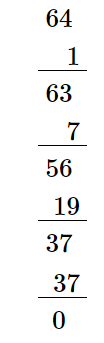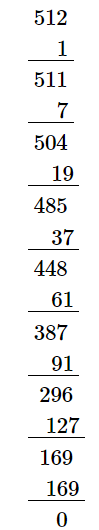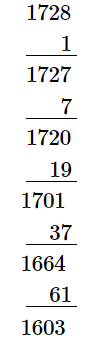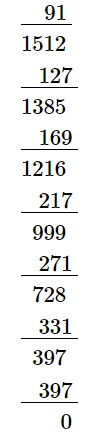# Find the cube roots of the following numbers by successive subtraction of numbers:`
Question:

Find the cube roots of the following numbers by successive subtraction of numbers:

1, 7, 19, 37, 61, 91, 127, 169, 217, 271, 331, 397, ...

(i) 64

(ii) 512

(iii) 1728

Solution:

(i)
We have:$\because$ Subtraction is performed 4 times.

$\therefore \sqrt{64}=4$

(ii)
We have:$\because$ Subtraction is performed 8 times.

$\therefore \sqrt{512}=8$

(iii)
We have:$\because$ Subtraction is performed 12 times.

$\therefore \sqrt{1728}=12$